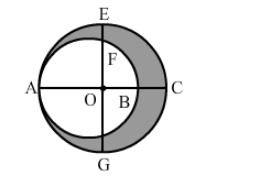# In the given figure, O is the centre of the bigger circle, and AC is its diameter. Another circle with AB as diameter is drawn.`
Question:

In the given figure, O is the centre of the bigger circle, and AC is its diameter. Another circle with AB as diameter is drawn. If AC = 54 cm and BC = 10, find the area of the shaded region.Solution:

We have:
OA = OC = 27 cm

$A B=A C-B C$

$=54-10$

$=44$

AB is the diameter of the smaller circle.
Thus, we have:

Radius of the smaller circle $=\frac{\mathrm{AB}}{2}=\frac{44}{2}=22 \mathrm{~cm}$

Area of the smaller circle $=\pi r^{2}$

$=\frac{22}{7} \times 22 \times 22$

$=1521.14 \mathrm{~cm}^{2}$

Radius of the larger circle $=\frac{\mathrm{AC}}{2}=\frac{54}{2}=27 \mathrm{~cm}$

Area of the larger circle $=\pi \mathrm{r}^{2}$

$=\frac{22}{7} \times 27 \times 27$

$=2291.14 \mathrm{~cm}^{2}$

∴ Area of the shaded region = Area of the larger circle -">Area of the smaller circle

$=2291.14-1521.14$

$=770 \mathrm{~cm}^{2}$